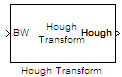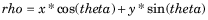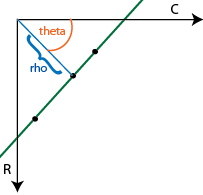# Hough Transform

Find lines in images

## Library

Transforms

`visiontransforms`

•## Description

Use the Hough Transform block to find straight lines in an image. The block outputs the Hough space matrix and, optionally, the rho-axis and theta-axis vectors. Peak values in the matrix represent potential straight lines in the input image. Generally, the Hough Transform block precedes the Hough Lines block which uses the output of this block to find straight lines in an image. You can instead use a custom algorithm to locate peaks in the Hough space matrix in order to identify potential straight lines.

PortInput/OutputSupported Data TypesSupported Complex Values

BW

Matrix that represents a binary image

Boolean

No

Hough

Parameter space matrix

• Double-precision floating point

• Single-precision floating point

• Fixed point (unsigned, fraction length equal to 0)

• 8-, 16-, 32-bit unsigned integer

No

Theta

Vector of theta values

• Double-precision floating point

• Single-precision floating point

• Fixed point (signed)

• 8-, 16-, 32-bit signed integer

No

Rho

Vector of rho values

Same as Theta port

No

## Parameters

Specify the spacing of the Hough transform bins along the theta-axis.

Rho resolution (pixels)

Specify the spacing of the Hough transform bins along the rho-axis.

Output theta and rho values

If you select this check box, the Theta and Rho ports appear on the block. The block outputs theta and rho-axis vector values at these ports.

Output data type

Specify the data type of your output signal.

Rounding mode

Select the rounding mode for fixed-point operations.

Overflow mode

Select the overflow mode for fixed-point operations.

Sine table

Choose how to specify the word length of the values of the sine table:

• When you select `Binary point scaling`, you can enter the word length of the sine table values, in bits.

• When you select `Slope and bias scaling`, you can enter the word length of the sine table values, in bits.

The sine table values do not obey the Rounding mode and Overflow mode parameters; they always saturate and round to `Nearest`.

Rho

Choose how to specify the word length and the fraction length of the rho values:

• When you select `Binary point scaling`, you can enter the word length and the fraction length of the rho values, in bits.

• When you select `Slope and bias scaling`, you can enter the word length, in bits, and the slope of the rho values. All signals in Computer Vision Toolbox™ blocks have a bias of 0.

Product output

. Use this parameter to specify how to designate the product output word and fraction lengths:

• When you select `Binary point scaling`, you can enter the word length and the fraction length of the product output, in bits.

• When you select `Slope and bias scaling`, you can enter the word length, in bits, and the slope of the product output. All signals in Computer Vision Toolbox blocks have a bias of 0.

See Multiplication Data Types for illustrations depicting the use of the product output.

Accumulator

Use this parameter to specify how to designate this accumulator word and fraction lengths:

• When you select `Same as product output`, these characteristics match the characteristics of the product output.

• When you select `Binary point scaling`, you can enter the word length and the fraction length of the accumulator, in bits.

• When you select `Slope and bias scaling`, you can enter the word length, in bits, and the slope of the accumulator. All signals in Computer Vision Toolbox blocks have a bias of 0.

See Multiplication Data Types for illustrations depicting the use of the accumulator data type in this block.

Lock data type settings against change by the fixed-point tools

Select this parameter to prevent the fixed-point tools from overriding the data types you specify on the block mask. For more information, see `fxptdlg` (Fixed-Point Designer), a reference page on the Fixed-Point Tool in the Simulink® documentation.

Hough output

Choose how to specify the word length and fraction length of the Hough output of the block:

• When you select `Binary point scaling`, you can enter the word length of the Hough output, in bits. The fraction length always has a value of 0.

• When you select `Slope and bias scaling`, you can enter the word length, in bits, of the Hough output. The slope always has a value of 0. All signals in Computer Vision Toolbox blocks have a bias of 0.

Theta output

Choose how to specify the word length and fraction length of the theta output of the block:

• When you select `Binary point scaling`, you can enter the word length and the fraction length of the theta output, in bits.

• When you select `Slope and bias scaling`, you can enter the word length, in bits, and the slope of the theta output. All signals in Computer Vision Toolbox blocks have a bias of 0.

## Algorithm

The Hough Transform block implements the Standard Hough Transform (SHT). The SHT uses the parametric representation of a line:The upper-left corner pixel is assumed to be at x=0,y=0.

The variable rho indicates the perpendicular distance from the origin to the line.

The variable theta indicates the angle of inclination of the normal line from the x-axis. The range of theta is $-\frac{\pi }{2}\le \theta <+\frac{\pi }{2}$ with a step-size determined by the Theta resolution (radians) parameter. The SHT measures the angle of the line clockwise with respect to the positive x-axis.

The Hough Transform block creates an accumulator matrix. The (rho, theta) pair represent the location of a cell in the accumulator matrix. Every valid (logical true) pixel of the input binary image represented by (R,C) produces a rho value for all theta values. The block quantizes the rho values to the nearest number in the rho vector. The rho vector depends on the size of the input image and the user-specified rho resolution. The block increments a counter (initially set to zero) in those accumulator array cells represented by (rho, theta) pairs found for each pixel. This process validates the point (R,C) to be on the line defined by (rho, theta). The block repeats this process for each logical true pixel in the image. The Hough block outputs the resulting accumulator matrix.

 Find Local Maxima Computer Vision Toolbox Hough Lines Computer Vision Toolbox `hough` Image Processing Toolbox™ `houghlines` Image Processing Toolbox `houghpeaks` Image Processing Toolbox